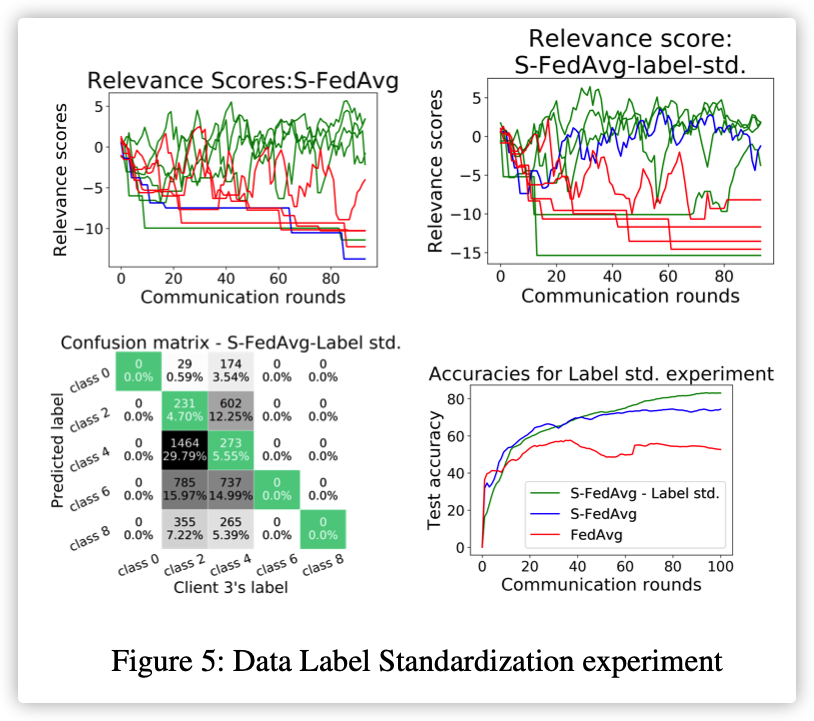AAAI Conference on Artificial Intelligence

## 0x00 Abstract

Though FL paradigm has received significant interest recently from the research community, the problem of selecting the relevant clients w.r.t. the central server’s learning objective is under-explored. We refer to these problems as Federated Relevant Client Selection (FRCS). Because the server doesn’t have explicit control over the nature of data possessed by each client, the problem of selecting relevant clients is significantly complex in FL settings.

The problems of FRCS need to be resolved:

• selecting clients with relevant data
• detecting clients that possess data relevant to a particular target label
• rectifying corrupted data samples of individual clients.

We follow a principled approach to address the above FRCS problems and develop a new federated learning method using the Shapley value concept from cooperative game theory. Towards this end, we propose a cooperative game involving the gradients shared by the clients. Using this game, we compute Shapley values of clients and then present Shapley value based Federated Averaging (S-FedAvg) algorithm that empowers the server to select relevant clients with high probability.

## 0x01 Introduction

FL setting assumes a syn- chronous update of the central model and the following steps proceed in each round of the learning process (McMahan et al. 2017).

Note that only a fraction of clients is selected in each round as adding more clients would lead to diminishing returns beyond a certain point.

Though initially the emphasis was on mobile- centric FL applications involving thousands of clients, recently there is significant interest in enterprise driven FL applications that involve only a few tens of clients.

### Motivation:

We apply standard FedAvg algorithm to two cases:

(a) where all clients possess relevant data

(b) where some clients possess irrelevant data.

NOTE：将奇数标签改成偶数！

To simulate irrelevance, we work with open-set label noise (Wang et al. 2018) strategy, wherein we randomly flip each odd label of the 4 irrelevant clients to one of the even labels.

### Contributions of Our Work:

• 将夏普利值作为衡量设备数据相关性的度量
• 基于夏普利值魔改FedAvg算法(S-FedAvg)
• 解决了两个FRCS问题：
• 选择高质量数据的节点
• 检测和纠正节点的异样数据

## 0x03 Problem Statement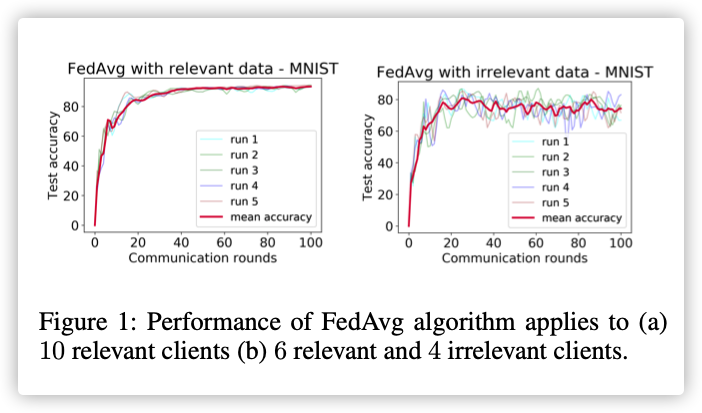## 0x04 Proposed Solution

### 博弈基础

$SV_i(v)=\frac{1}{|N|!}\sum_{\pi \in \Pi}[v(C_{\pi}(i)\cup\{i\})-v(C_{\pi}(i))]$

$SV_i(v)=\sum_{C \in A-\{i\}}\frac{|C|!(|N|-|C|-1)!}{|N|!}[v(C(i)\cup\{i\})-v(C(i))]$

$\{2,3,1,4,5,6\}\\ \{2,3,1,4,6,5\}\\ ...\\ \{3,2,1,4,5,6\}\\ \{3,2,1,4,6,5\}\\ ...\\$

### 相关度得分的计算流程：

• 训练节点$k$将参数更新$\delta^{t+1}_k=\theta^{t+1}_k-\theta^t$发送至服务器；

• 定义合作游戏$(\delta^{t+1},v)$,其中$\delta^{t+1}=\{\delta^{t+1}_s\}_{s\in S^t}$,$v(\cdot)$是特征函数；

$\theta^{t+1}_{X}=\theta^t+\frac{1}{|X|}\sum_{s \in X}\delta^{t+1}_s\\ v(X,D_V)=\mathcal{P}(f_{\theta^{t+1}_X},D_V)$

$\mathcal{P}(,)$代表中央模型参数$\theta^{t+1}_X$在验证数据集$D_V$上的性能.

• $\varphi=(\varphi_1,\varphi_2,...,\varphi_K)$表示相关度向量，其中$\varphi_k$表示$Client_k$的相关度。（$\varphi$只保留在服务器,初始化为$\varphi_k=\frac{1}{K}$)

We posit that more the relevance value for a client, more is its likelihood to be relevant and thereby more is its contribution to the objective of central model.

• 每轮聚合时，从合作游戏中获得夏普利值$sv(s),\forall s\in S_t$,更新相关度向量：

$\varphi_s=\alpha*\varphi_s+\beta*sv(s);\forall s \in S_t$

• 使用$softmax(\cdot)$计算相对相关度$P_k$，依据$P_k$选取参与训练节点集$S^t$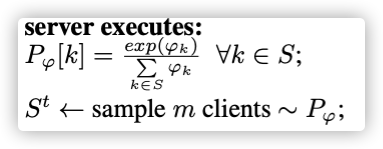### 具体算法：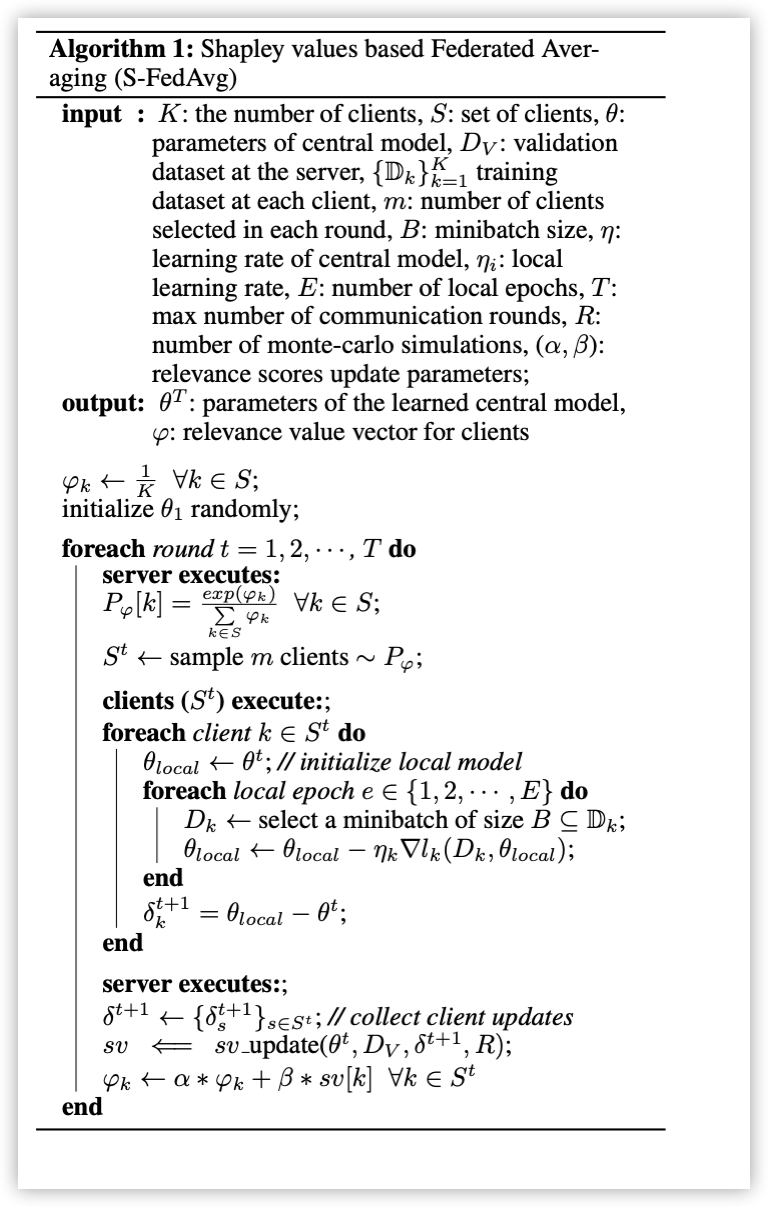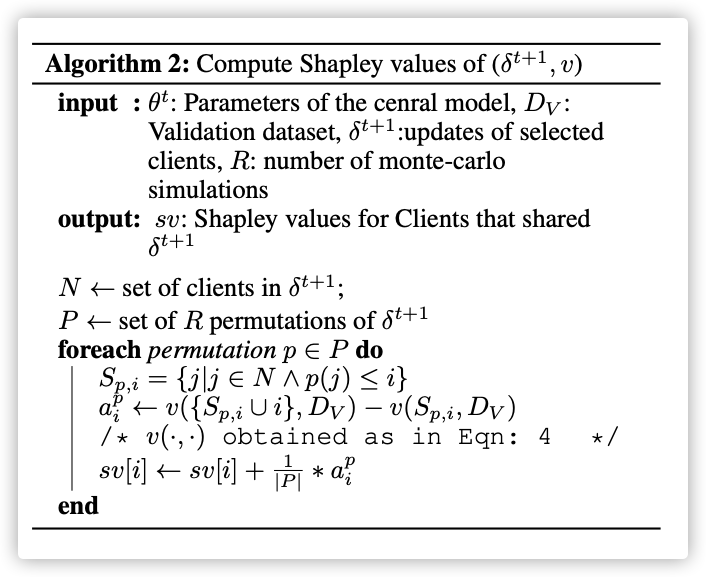## 0x05 Experiments

### 1.Setup

• Extreme 1-class-non-iid

As is typical with the federated learning setting, we assume that the data is distributed non-iid with each client. We follow the extreme 1-class-non-iid approach mentioned in (Zhao et al. 2018b) while distributing the data to clients.

• $|D_V|=1000,|D_{test}|=4000$

• 验证无关数据的干扰，改变了奇数的label，规则如下：

$k=\{1→0,5→2,3→4,9→6,7→8\}$

• Hyper-parameter Values: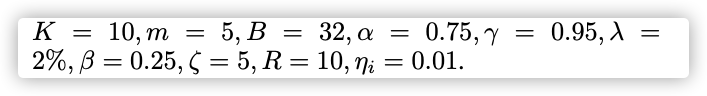### 2.S-FedAvg: To Selects Relevant Clients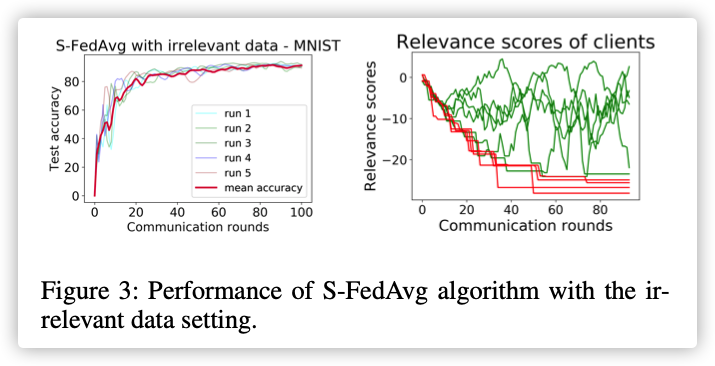### 3.Class-specific Best Client Selection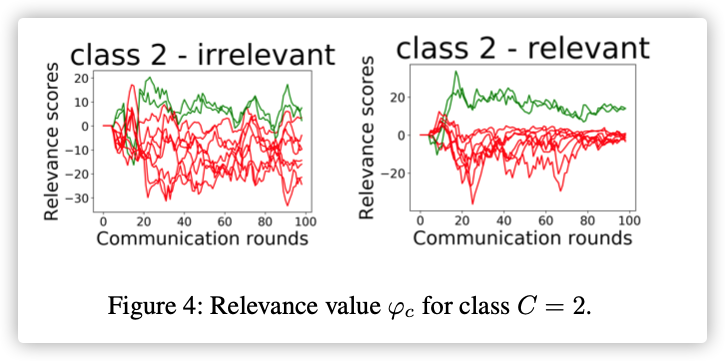### 4.Data Label Standardization